Electricity & Megnetism

# Electric current

Electric current is the physical phenomenon in which electric charge carriers, such as electrons, are conducted through the interior of some material due to the application of an electric potential difference . The electrical conduction capacity of materials depends directly on the intensity of the electrical potential applied to them, as well as on their nature: while conducting materials are easily traversed by electrical currents, insulating materials offer great resistance to them.

Electric current is a scalar quantity. Its unit of measurement, according to the International System of Units, is the ampere (A). This unit measures the magnitude of the electric charge that crosses the cross section of a conductor every second and, therefore, can also be written as coulombs per second (C/s).

## Electric current formula

The electric current is defined by the ratio between the magnitude of the electric charge that passed through the conductor and the time interval in which this electric current was maintained. Check the electric current formula below: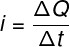i – electric current (A)

ΔQ – electrical charge (C)

Δt – time interval (s)

It is possible to relate the electric current to the number of electrons that pass through a conducting body during a given time interval. Watch: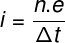n – number of electrons

e – fundamental electrical charge (e = 1.6 x 10-19 C )

## Conventional direction and real direction of electric current

Electric current in conductors occurs through the movement of electrons. These, in turn, have charges of negative sign, for this reason, when conducted, they always walk towards the positive (higher) electrical potential. This direction of electric current is known as the real direction .

For reasons of simplification, the conventional direction of the electric current is adopted. In the conventional sense , we assign the positive sign to the charge on the electrons , so the electrons must always move toward the negative (lower) pole .

The reason for using this convention is intuitive : when electrons move inside conductors, their electrical potential must drop, since, due to frequent collisions with atoms, they dissipate part of their energy during their conduction.

### In short:

The movement of electrons occurs as follows:

• Real sense: lower potential → higher potential
• Conventional meaning: higher potential → lower potential

Types of electric current

There are two types of electric current: direct current and alternating current. Direct current is one in which electrons move in only one direction. This type of current is common in devices that use low voltages , such as electronics in general.

Alternating current is characterized by the oscillatory motion of electrons. In this type of current, a rapid polarity reversal of the electrical potential causes electrons to move back and forth around a fixed position. Alternating electric current is mainly used in electric motors and in the transmission of electricity: the electric current that reaches our homes, made available at outlets, is an alternating electric current.

The transmission of electricity is done in alternating current due to the advantage that this type of current presents in relation to direct current. Due to the shortest path taken by the electrons, the Joule effect losses are smaller when using alternating current.

## Effects of electric current

The passage of electric current can cause several different effects according to the medium that conducts it. Check out some of the most common effects:

• Thermal effect: When the electric current encounters some resistance to its passage, it causes heating, due to the Joule effect.
• Magnetic effect: Conductors crossed by an electric current produce magnetic fields, as in the case of the coils used in artificial magnets.
• Luminous effect: When a conductor undergoes great heating, it is possible that it starts to emit visible light, as in the case of incandescent lamps.

## Electric current and electric power

From the intensity of the electric current and the electrical resistance of some material, it is possible to calculate the amount of heat that is dissipated due to the Joule effect. Check the formulas that are used to calculate the dissipated power: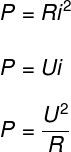P – electrical power (W)

R – electrical resistance (Ω)

i – electric current (A)

U – electrical voltage (V)

## Conductors and insulators

Materials that are good conductors , such as transition metals (copper, silver, gold, platinum, for example), have large numbers of electrons that are weakly attracted to their atomic nuclei. This property allows these electrons to move inside the metals with relative ease, being able to be conducted only by the application of a small potential difference.

Insulating materials offer great resistance to the flow of electric current , mainly due to the low number of free electrons available for conduction. In order for electric currents to form in this type of materials, it is necessary to apply electrical potentials of very high intensities, until the medium starts to behave like a conductor. In this case, we say that a dielectric breakdown has occurred .

## Exercises solved on electric current

Question 1) Determine the magnitude of the electric current flowing through a conducting wire knowing that, during a time interval of 2.0 s, this conductor was traversed by a total of 4.0 x 10 20 electrons.

a) 1.6 A

b) 3.2 A

c) 16 A

d) 32 A

e) 20 A

Template: Letter D

Resolution:

To solve this exercise, we will use the formula for quantized electric current.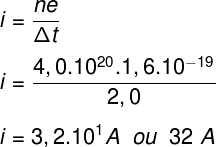According to the calculation performed, the electric current in the wire has an intensity of 32 A.

Question 2) According to the conventional sense of electric current, we can say that:

a) electrons only move due to thermal agitation.

b) electrons move from lower to higher potential.

c) electrons move from higher to lower potential.

d) electrons move in oscillatory back and forth motions.

e) electrons remain in the vicinity of atomic nuclei.

Template: Letter C

Resolution:

According to the conventional direction of electric current, electrons move from the higher electric potential towards the lower electric potential. Therefore, the correct alternative is letter C.

Question 3) Calculate the magnitude of electric charge that passes through a wire carrying an electric current of 3.0 A during a time interval of 2.0 s.

a) 3.0 C

b) 6.0 C

c) 1.5 C

d) 0.5 C

e) 2.0 C

Template : Letter B

Resolution :

To calculate the magnitude of the electric charge, it is necessary to use the electric current formula. Watch: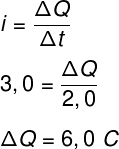According to the calculation and the exercise data, during the time interval of 2.0 s, an electrical charge of 6.0 C passed through the wire.